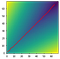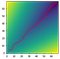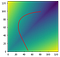# Gradient Descent and Chain-Linked Systems

On Broken Incentives and Deadlock.

By Shawn Jain and Blake Elias
[Shawn Jain is an AI Resident at Microsoft Research.
Blake Elias is a Researcher at the New England Complex Systems Institute.]

# Origins

There are portions of organizations, and even of economies, that are chain-linked. When each link is managed somewhat separately, the system can get stuck in a low-effectiveness state. That is, if you are in charge of one link of the chain, there is no point in investing resources in making your link better if other link managers are not.

To make matters even more difficult, striving for higher quality in just one of the linked units may make matters worse! Higher quality in a unit requires investments in better resources and more expensive inputs, including people. Since these efforts to improve just one linked unit will not improve the overall performance of the chain-linked system, the system’s overall profit actually declines. Thus, the incentive to improve each unit is dulled. 

We wondered, how can this idea be made computational? If an organization were run by one or more AI agents, would it be capable of getting past these limitations? This issue is connected, of course, to game-theoretic notions of social dilemmas and mechanism design.

Our intuition is that computation and AI should be able to solve problems where fragmented human organizations get stuck. On the other hand, today’s AI is quite brittle. One failure mode is the existence of local optima. To what extent can a social dilemma (i.e. a sub-optimal equilibrium in a game) be seen as a local optimum in some learning algorithm (where the learning algorithm consists of the behavior of the agents)?

# Problem Setup

The owner wants to earn as much profit as possible. Trying to align incentives, the owner tries tying station managers’ bonus to the factory’s profits. The owner hopes to optimize the rate of production at each station to maximize his profit. (Note: assume the cost per widget at each station is fixed.)

The managers can raise or lower only their station’s output, incurring a cost of \$𝛼 or \$β per widget. They do not coordinate.

But there’s one problem. If the manager of station A increases his station’s output, while station B’s output remains the same, the firm actually loses money. This is because station A spent more to increase its output, but the factory overall produced the same total number of widgets (because station B couldn’t keep up). This is what the author calls a “chain-linked system”, because the output is determined by the weakest link. If Station B is behind, there’s no point in Station A increasing its output — in fact, that would bring a net loss to the firm. Stations A and B have to improve jointly in order for profits to increase. But as a manager, if you only direct your single station, you’re not incentivized to improve at all (since you’re just spending the company’s money with no return) — and so deadlock occurs.

Could the owner set production rates directly instead of relying on this bonus plan? Let’s see if optimization can solve this problem.

Define the following variables:

• a: the output of station A
• b: the output of station B
• 𝛼: cost per unit output of station A
• β: cost per unit output of station B
• ɣ: sale price for a completed widget

We produce min(a, b) completed widgets (since every widget requires a unit of output from both stations A and B). Since we sell each widget for a price of ɣ, we will bring in revenue according to:

R(a, b) = min(a, b) * ɣ,

Our cost will be:

C(a, b) = a * 𝛼 + b * β

We are then trying to maximize profit:

P(a, b) = R(a, b) - C(a, b) = min(a, b) * ɣ - [a * 𝛼 + b * β]

We then define a “loss” function,

L(a, b) = -P(a, b)

to convert the problem into a minimization that can be solved with standard optimizers (e.g. gradient descent).

Note that increasing a alone, or b alone, decreases profit. But, if we increase a and b together, profit increases. Consider the gradient dL/da. This assumes all variables except a are held constant. The same holds for dL/db — we only get to vary b. However, we need to increase both a and b simultaneously to improve the loss function. Since SGD is based on gradient computations only, will it successfully optimize in this scenario? In other words, how does SGD deal with co-varying parameters?

This is also not a traditional supervised learning problem; although we have two input variables, a and b, we do not have a target output variable. We simply seek to minimize the loss by setting a and b to the best values. Therefore, this is purely an optimization problem — more along the lines of an operations research (OR) problem than a machine learning (ML) problem.

# Experimental Setup

Compute gradients using autograd & implement SGD from scratch:

Our notebook is here.

# ResultsFigure 1. Trajectory when Initialized to (a, b) = (1, 1)Figure 2. Trajectory when Initialized to (a, b) = (3, 5). [Note that the Adam optimizer would prevent the ‘waviness’ because momentum would ‘cancel out’ the lateral movement.]Figure 3. Trajectory when Initialized to (a, b) = (50, 2). Notice the non-intuitive behavior — SGD simultaneously decreases a while increasing b. Figures 1 and 2 match our intuitions more closely — simultaneously increasing a and b.

# Discussion

What would gradient descent do?
To remind ourselves, we are optimizing:

where:

The gradients are:

What about when a = b = 1: will there be deadlock? The equations above would give dL/da = 𝛼 = 1 and dL/db = β = 1. This would indicate that we should decrease both a and b by the same amount, since we step in the direction of the negative gradient. If we were to do this, we would see a and b continually shrink together, and eventually both reach 0.

What would PyTorch do?

PyTorch’s evaluation on (a, b) = (1, 1) gives dL/da = 1 (i.e. dL/da = 𝛼, as expected). But, it gives dL/dB= -2 (i.e. β - ɣ, taken from the b < a case, when in fact b = a). Although torch.min(a, b) is not technically differentiable, PyTorch ‘breaks the tie’ — avoiding the deadlock scenario.

What would we do intuitively?

How would we intuitively solve this problem? Suppose we start at v = <a, b> = <1, 1>. The best possible update would seem to be: v’ = v + u*<1, 1> (increase a and b equally). Let’s compute the directional derivative from this update:

Does this match gradient descent?

Let’s compare our intuitive answer with our gradient descent calculation. Using an equation for the directional derivative, we would hope to have:

We only have this guarantee when L is differentiable at v = <a, b>. But when a = b, L is not technically differentiable. However, if we use the “tie-breaking” that PyTorch does, we get the following:

which indeed holds.

While the components of L (i.e., dL/da and dL/db) both have a case-analysis embedded in their definition, we note that their sum is always 𝛼 + β - ɣ, as long as a ≠ b. And with PyTorch’s implementation for the gradient of the min function, we get dL/da + dL/db = 𝛼 + β - ɣ everywhere, even when a = b.

We knew ahead of time, that jointly increasing a and b (i.e. v’ = v + u*<1, 1>) was the best direction to step in, given a = b. And the derivation above shows that taking separate gradients with respect to a and b, then linearly combining these gradients, achieves the same result. This is why, even though the optimizer operates in the (a, b) space, it can correctly optimize this problem.

Why can’t the managers in our story solve this problem? Let’s say each manager computes his gradient; dL/da or dL/db. Can they simply each take a step according to their gradient? The managers get stuck in the “otherwise” case shown in the equations for dL/da and dL/db — i.e., where a = b. PyTorch has symmetry-breaking which solves this problem. But this does not map cleanly to the motivating problem.

Note: We have not considered alternate initializations (i.e. a ≠ b), nor how the direction of the derivative changes with different 𝛼 and β values (Hint: SGD will take a step in the <𝛼, β> direction iff a = b).

An unanswered question: Over the course of a single trajectory (e.g. Figure 2), why does the period of the wave appear to grow larger?

# Future Work & Conclusion

## Trajectory & Connection to RL

In the current set-up, we are optimizing for profit/loss without consideration for inventory (time dimension). What about changing the trajectory to temporarily over-produce to work through accumulated inventory and maximize profit over time?

# Footnotes

For instance, the various problems at General Motors from 1980 to 2008 had strong chain-link features. Increasing the quality of an automobile transmission does little good if the knobs fall off the dashboard and door panels continue to rattle. Improving fit and finish, along with the drivetrain, may offer little overall improvement as long as the designers continue to produce pedestrian designs. Improving the look of the automobiles may only increase costs unless the complex technology of design for manufacturability is mastered. And so on.